# Texas Go Math Grade 2 Lesson 3.2 Answer Key Different Forms of 4-Digit Numbers

Refer to our Texas Go Math Grade 2 Answer Key Pdf to score good marks in the exams. Test yourself by practicing the problems from Texas Go Math Grade 2 Lesson 3.2 Answer Key Different Forms of 4-Digit Numbers.

## Texas Go Math Grade 2 Lesson 3.2 Answer Key Different Forms of 4-Digit Numbers

Explore

Write how many thousands, hundreds, tens, and ones. Write the number.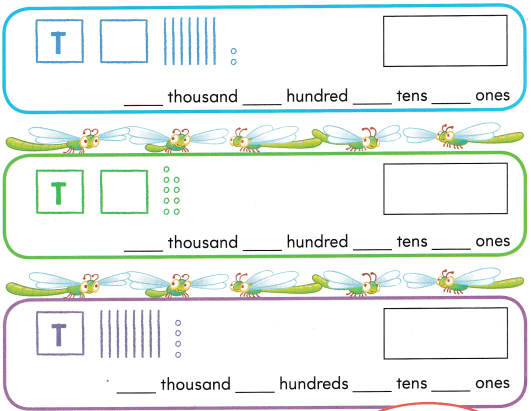FOR THE TEACHER • Read the following problem. Derek drew quick pictures to show three numbers. What numbers did Derek show?Explanation:
Derek draws the quick pictures like,
box with T represents thousands,
empty box represent hundreds,
bar sticks represents tens and
circles represents ones.
So, it is easy to write the numbers  by seeing the pictures.

Math Talk
Mathematical Processes

Explain Why it is important to write the zero in the number 1084.
It is important to write zero in the number 1084 as there is no number in the hundreds place and
the numbers of digits in that number must and should be four digit number.

Model and Draw

These are different ways to write a 4-digit number.
one thousand, one hundred thirty-five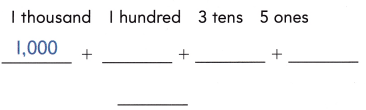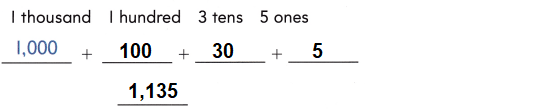Explanation:
As, we know that ten hundreds is 1 thousand,
10 tens is one hundred,
ten ones is one ten and the number in units place refers to ones.

Share and Show

Write the number in different ways.

Question 1.
one thousand, two hundredExplanation:
In the above question there are 10 hundreds in 1 thousand.
There are 2 hundreds in 200.
Where as in tens and ones place there is zero.
So, the number is 1,200

Question 2.
one thousand eighteen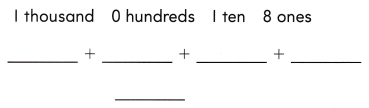Explanation:
In the above question there are 10 hundreds in 1 thousand.
There is zero in hundreds place.
There are 10 ones in 1 ten and 8 ones.
So, the number is 1,018

Question 3.
one thousand, one hundred thirty-twoExplanation:
In the above question there are 10 hundreds in 1 thousand.
There are 10 tens in 100.
3 tens in 30 and 2 ones..
So, the number is 1,132

Problem Solving

Write the number in different ways.

Question 4.
one thousand, one hundred seventyExplanation:
In the above question there are 10 hundreds in 1 thousand.
There are 10 tens in 100.
There are 7 tens in 70
So, the number is 1,170

Question 5.
one thousand, one hundred eight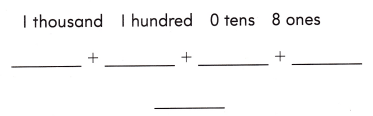Explanation:
In the above question there are 10 hundreds in 1 thousand.
There are 10 tens in 100 and 8 ones.
put zero in tens place to represent the place value as there is no number in tens place.
So, the number is 1,108

Question 6.
H.O.T. Terry wrote to show a number. Draw quick pictures to show Terry’s number. Write the number.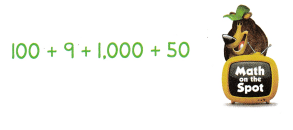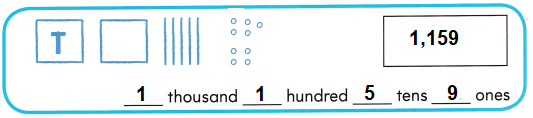Explanation:
In the above question there are 10 hundreds in 1 thousand.
There are 10 tens in 100.
5 tens in 50 and 9 ones
So, the number is 1,159

Question 7.
H.O.T. Multi-Step Manny writes a 4-digit number.

• The number has I thousand.
• The hundreds digit is less than the thousands digit.
• The sum of the tens and ones digits is 10.

What number could May write?
Explanation: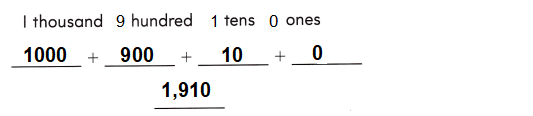Question 8.
Representations Kimmie writes this number. Which is another way to write this number?
1 thousand, 1 hundred, 3 tens, and 2 ones
(A) 1,122
(B) 232
(C) 1,132
Option (C)
Explanation:
1000 + 100 + 30 + 2 = 1,132
So, there are 10 hundreds in 1 thousand.
There are 10 tens in 100.
3 tens in 30 and 2 ones.
So, the number is 1,132

Question 9.
Multi-Step Byron writes this number. Which is another way to write the number?
one thousand, forty-two
(A) 1,000 + 40 + 2
(B) 1,000 + 400 + 20
(C) 100 + 40 + 2
Option (A)
Explanation:
1000 + 40 + 2 = 1,042
So, there are 10 hundreds in 1 thousand.
4 tens in 40 and 2 ones,
hundred place is represented with zero.
So, the number is 1,042

Question 10.
TEXAS Test Prep At the Holland Corn Festival, 1,150 ears of corn were cooked for the corn eating contest. Which is another way to write 1,150?
(A) 1,000 + 100 + 50
(B) 100 +100 + 50
(C) 1,000 +10 + 5
Option (A)
Explanation:
1000 + 100 + 50 + 0 = 1,150
So, there are 10 hundreds in 1 thousand.
There are 10 tens in 100.
5 tens in 50
So, the number is 1,150

TAKE HOME ACTIVITY • Have your child explain how he or she solved one of the exercises in this lesson.
Child can do better after repeated practice of problems in the exercises in this lesson.
By drawing the quick pictures to represent the place values.

### Texas Go Math Grade 2 Lesson 3.2 Homework and Practice Answer Key

Write the number in different ways.

Question 1.
one thousand, seventy-three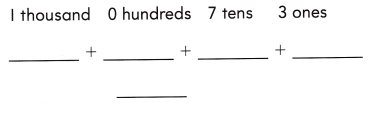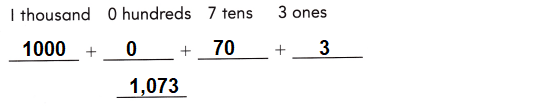Explanation:
1000 + 0 + 70 + 3 = 1,073
So, there are 10 hundreds in 1 thousand.
7 tens in 70 and 3 ones.
Zero in hundreds place.

Question 2.
one thousand, one hundred, sixty-eightExplanation:
1000 + 100 + 60 + 8 = 1,168
So, there are 10 hundreds in 1 thousand.
There are 10 tens in 100.
6 tens in 60 and 8 ones.
So, the number is 1,168

Problem Solving

Solve.

Question 3.
Wendy wrote to show a number. Draw quick pictures to show Wendy’s number. Write the number.
100 + 4 + 1,000 + 30Explanation:
1000 + 100 + 30 + 2 = 1,132
So, there are 10 hundreds in 1 thousand.
There are 10 tens in 100.
30 tens in 3 and 4 ones.
So, the number is 1,134

Lesson Check

Question 4.
Bart wrote to show a number. Which is another way to write Barfs number?
1 thousand, 0 hundreds, 8 tens, and 1 one
(A) 181
(B) 811
(C) 1,081
Option(C)
Explanation: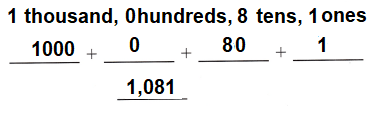Question 5.
Lee Ann wrote to show a number. Which is another way Lee Ann could write the number?
one thousand, fifty-six
(A) 1,000 + 50 + 6
(B) 1,000 + 500 + 6
(C) 100 + 50 + 6
Option (A)
Explanation:
The other way she can show by drawing quick pictures.Question 6.
Sam is making a quick picture for 1,159. He draws 9 ones, 5 tens, and 1 thousand. How many hundreds will he draw?
(A) 1
(B) 17
(C) 7